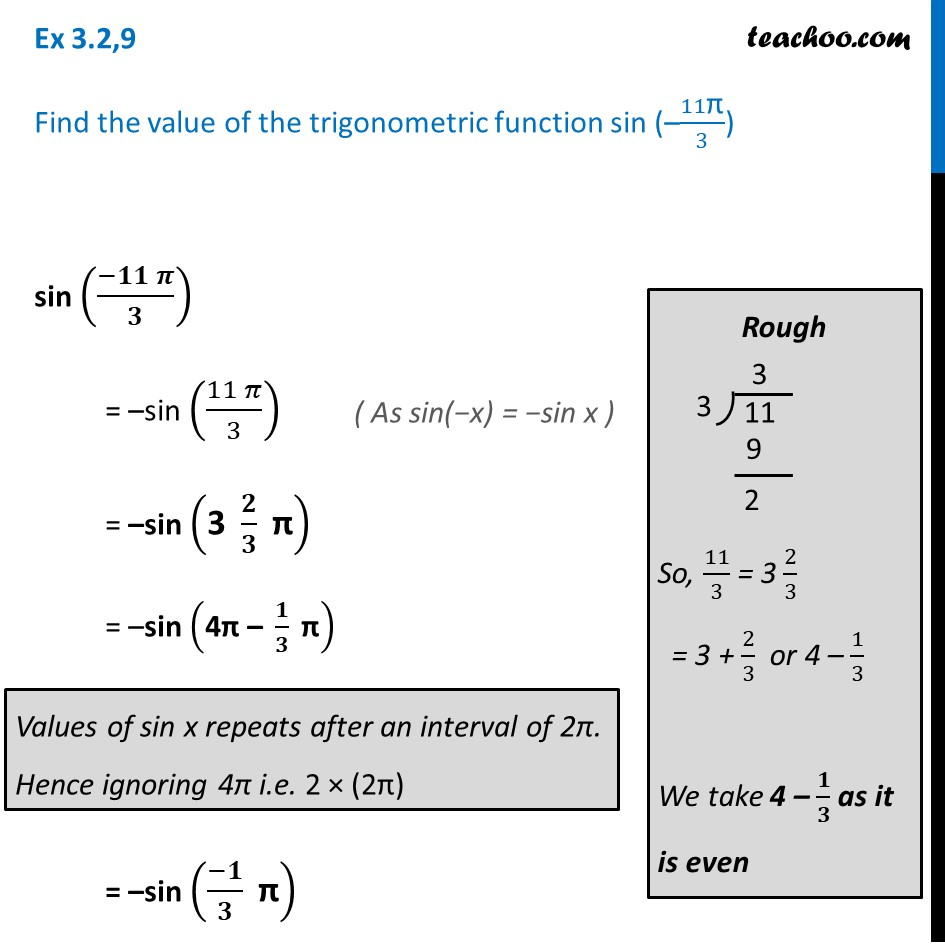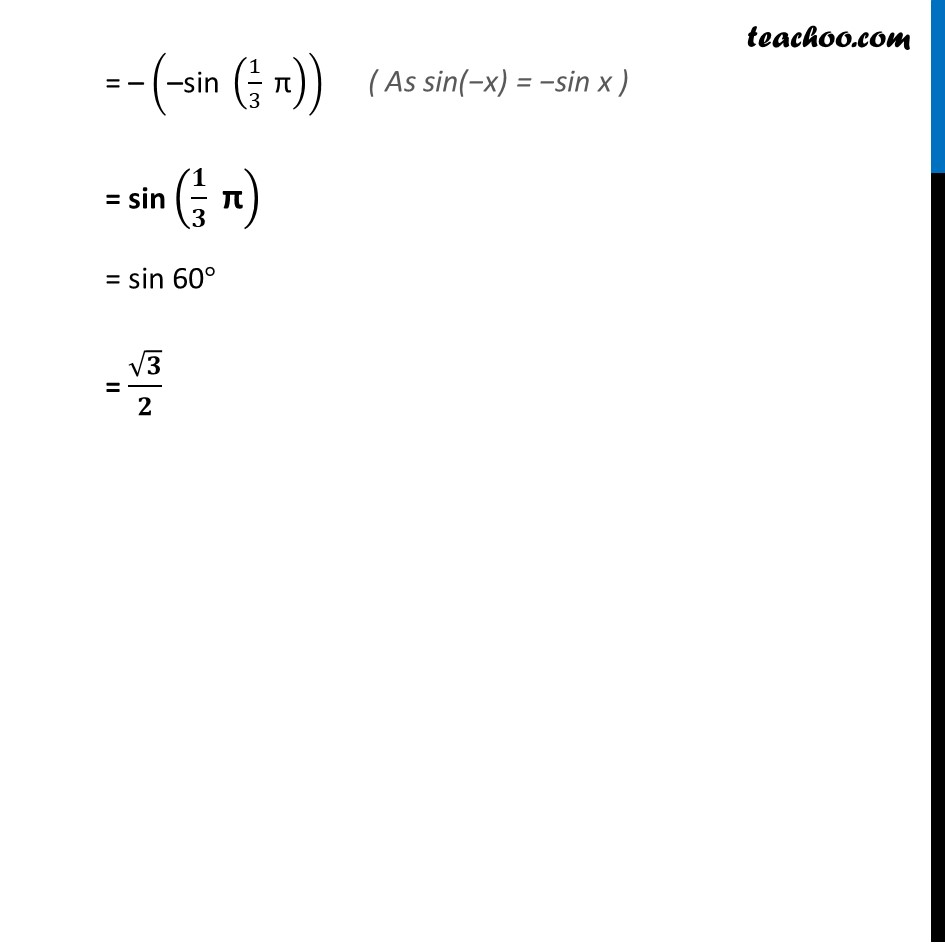Ex 3.2

Chapter 3 Class 11 Trigonometric Functions
Serial order wiseLearn in your speed, with individual attention - Teachoo Maths 1-on-1 Class

### Transcript

Ex 3.2,9 Find the value of the trigonometric function sin (–11"π" /3) sin ((−𝟏𝟏 𝝅)/𝟑) = –sin ((11 𝜋)/3) = –sin ("3 " 𝟐/𝟑 " π" ) = –sin ("4π – " 𝟏/𝟑 " π" ) = –sin ((−𝟏)/𝟑 " π" ) Rough So, 11/3 = 3 2/3 = 3 + 2/3 or 4 – 1/3 We take 4 – 𝟏/𝟑 as it is even = – ("–sin " (1/3 " π" )) = sin (𝟏/𝟑 " π" ) = sin 60° = √𝟑/𝟐